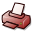# Axiomatizations of Compositional Inductive-Recursive Definitions

## DSpace Repository

 URI: Dokumentart: Dissertation Date: 2018-11-07 Language: English Faculty: 7 Mathematisch-Naturwissenschaftliche Fakultät Department: Informatik Advisor: Schroeder-Heister, Peter (Prof. Dr.) Day of Oral Examination: 2018-10-25 DDC Classifikation: 004 - Data processing and computer science100 - Philosophy510 - Mathematics Keywords: Typentheorie , Kategorientheorie , Konstruktive Mathematik , Kompositionalität , Semantik , Intuitionistische Mathematik Other Keywords:type theorycategory theorysemanticscompositionalityintuitionistic mathematicsconstructive mathematicspredicativity License: Order a printed copy:Print-on-Demand Show full item record

### Abstract:

\emph{Induction-recursion} is a definitional principle in Martin-L{\"o}f Type Theory defining families $(\U , \T: \U\to D) : \Fam{D}$ where $D: \Setone$ is an arbitrary fixed (large) set (which in motivating examples is chosen to be $D=\Set$), and $\U : \Set$ is defined by induction while $\T$ is simultaneously defined by recursion on $\U$; the qualifier simultaneously'' means here that $\U$ may depend on values of the function $\T : \U \to D$. Two equivalent\footnote{These axiomatizations are equivalent if the underlying logical framework is chosen appropriately.} axiomatizations of this situation were proposed by Dybjer-Setzer in \cite{dybjer99}\cite{dybjer03}. In both cases a (large) set of \emph{codes} $\DS\; D\; D$ (respectively $\DS'$) for inductive-recursive definitions is defined such that each code $c : \DS\; D\; D$ decodes to an endofunctor $\sem{c} : \Fam{D}\to \Fam{D}$ between categories of families whose initial algebra is the family defined by this code $c$. The authors proved the consistency of their axiomatizations be giving a set-theoretic model. These axiomatizations $\DS$ and $\DS'$ are however not the only reasonable axiomatizations of induction-recursion. There are at least two ways to come to this conclusion: the more practical one is motivated by the observation that while in the reference theory of inductive definitions it is always possible to compose (in a semantically sound way) two inductive definitions to a single new one, this seems hardly to be the case for the preexisting axiomatizations of Induction-Recursion. The second-, more conceptual observation about the existing axiomatizations of induction-recursion is that it does not contain constructors for dependent products (or powers\footnote{There is a close relationship between dependent products-, and powers of codes.}) of codes but only for dependent sums of codes. Indeed, we show that these two observations are related by characterizing compositionality of Dybjer-Setzer induction-recursion in terms of the existence of powers of codes by sets. Departing from this characterization, we define- and explore two new axiomatizations of induction-recursion satisfying the mentioned characterization and for which we prove compositionality. In the first one, this is achieved by restricting to a subsystem\footnote{By subsystem'' we mean here that there is a semantics-preserving translation from $\UF$ to $\DS$.} $\UF$ of $\DS$ of \emph{uniform codes} for which powers of codes exist. Consistency of this system is established by a semantics-preserving embedding into the system $\DS$. The second axiomatization $\IR$ of \emph{polynomial codes} (so called since they are based on the idea of iterating polynomials\footnote{Polynomials are also called \emph{containers} and are a formalization for inductive definitions.}) defines a system into which $\DS$ can be embedded and which contains a constructor for dependent products- (and in particular powers) of codes. While for $\UF$ the existence of a model can be obtained by embedding it into $\DS$ for which Dybjer-Setzer themselves devised a (set-theoretic) model, we cannot argue in this way for a model of $\IR$ and instead we provide a new model for the latter having almost the same set-theoretical assumptions concerning large cardinals: while Dybjer-Setzer induction-recursion can be modeled in ZFC supplemented by a Mahlo cardinal and a $0$-inaccessible, we need ZFC plus a Mahlo cardinal and a $1$-inaccessible. Since the system $\IR$ does not simply arise by adding a constructor for dependent products of codes to $\DS$ caeteris paribus, but additionally requires redefining all other constructors, the question about constructors generating the image of the inclusion $\DS\hookrightarrow \IR$ imposes itself; we approach this question by defining an intermediary system lying between $\DS$ and $\IR$ and give a translation of this intermediary system into $\DS$. This intermediary system does not only arise by removal of the constructor for dependent products of codes since this did not yet enable us to define a desired translation to $\DS$, but by introduction of an additional uniformity constraint realized by an annotation of codes by binary trees. A common feature of both new systems $\UF$ and $\IR$ is that they admit a more flexible relation between codes and their subcodes than this is the case for $\DS$: sub-$\DS$-codes of a given $\DS$-code do all have the same type while sub-$\UF$-, and sub-$\IR$-codes are not constrained in this way. This latter feature reveals a more abstract way of arriving at the conclusion that Dybjer-Setzer induction-recursion is not the most general formulation of induction-recursion: like inductive definitions can be characterized by a set of operations on sets\footnote{Theses operations are called stictly positive operations'' (see \cite{dybjer1996Wtypes}).}, inductive-recursive definitions are also determined by a set of operations on families (namely those represented by the set of functors defined by codes) and Dybjer-Setzer's systems did not take into account operations that change the (large) set $D$ indexing families while the new systems $\UF$ and $\IR$ do so. In line with the idea of considering induction-recursion as contributing to the pursue of the project of formalizing the meta theory of type theory in type theory itself \cite[p.1]{Dybjer96internaltype}, we return to Dybjer-Setzer's original formalization $\DS$ and provide a \emph{relationally-parametric} model for it that we articulate as a categories-with-families model in the category of reflexive graphs; categories-with-families were proposed in loc.cit. as a formalization of type theory inside type theory that is additionally well adapted to category theoretic reasoning. Relational parametricity is an established and important proof technique to establish meta-theoretic properties of type theories.